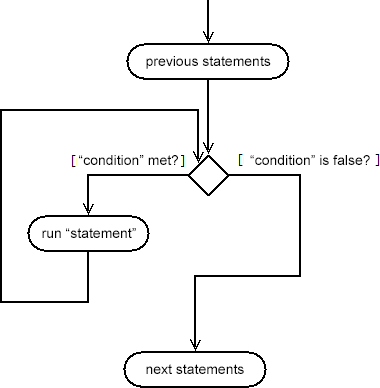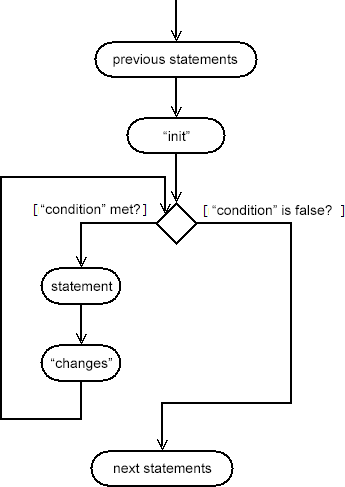## Control flow: loops

### Published on 2018-04-14

Loops enable you to define groups of statements that need to be executed several times (for example, while n<10). In C++, there are three important types of loops: while, do-while and for loops.

## The while statement

I believe that "while" is the simplest type of loop offered by the C++ programming language. The syntax of this statement is given below:

while (condition)
statement;Similar to the if statement, you need to define a "condition", as well as a "statement" (command) that needs to be executed (or a block of commands '{...}') when the condition is satisfied. But, unlike with the if statement, the command will be executed continuously - i.e. as long as the condition evaluates to true. Let's look at an example:

```#include <iostream>
using namespace std;

int main()
{
int i = 1;
int s = 0;

while (i <= 5)
{
cout << "Adding " << i << "." << endl;
s = s + i;

i++;                                        //increment the value of i
}

//1+2+3+4+5 = 15
cout << "Sum: " << s << endl;                    //prints 'Sum: 15'

return 0;
}
```

At the beginning, the variables i and s have the values 1 and 0, respectively. Then, because the condition (1 <= 5) is satisfied, the statements inside the block will be executed ("Adding 1." will be printed, the value of s will increase by i=1, and then the value of i will be incremented by 1). Now, instead of going to the statements that are below the block, the loop condition is checked. Because the condition (2 <= 5) is satisfied, the program executes the statements in the block again (prints "Adding 2.", increases s by i=2 and increments the value of i). Each of these runs (for i=1, i=2, etc) is called an "iteration" of the loop. The statements inside the block will be executed three more times (for i=3, i=4 and i=5), after which the condition (6 <= 5) will no longer be satisfied (6 <= 5 has the value false), and so the loop will terminate. Finally, the program will print the value inside the variable s - which represents the sum of the first 5 natural numbers 1+2+3+4+5=15.

If, during the first check, the condition has a value of false, then the statements inside the block will not execute (not even once). For example, in the program given above, if (in the beginning) the variable i received the value 10, then the condition in the while loop (10 <= 5) would have evaluated to false, and the program would not have entered the block - instead, it would have jumped to the end and print "Sum: 0".

It is very important (so don't forget!) to update the variable/variables that are part of the "condition" expression. Otherwise, the program will always perform the same statements, and it will never complete. It is your obligation to cause, at some point, a reason for the loop to end. In the program given above, this is done by increasing the value of the variable i. Since i is included in the condition expression (i <= 5), then at some point (when i gets a value greater than 5), the loop will end.

The following program demonstrates some of the things discussed above:

```#include <iostream>
using namespace std;

int main()
{
int z = 10;
while (z < 5)
{
cout << "This will never execute." << endl;
}

z = 6;
while (z >= 1)
{
cout << z << ", ";
z--;
}

cout << "END OF THE MATCH." << endl;
//output: 6, 5, 4, 3, 2, 1, END OF THE MATCH.

z = 100;
while (z < 1000)
{
//because 'z' is never updated, the condition
//(z < 1000) will always evaluate to true

//you have to kill this program (using the task manager)
//because it is now stuck printing the same number
cout << z << endl;
}

return 0;
}
```

## The for statement

If you know exactly how many times a statement (or a group of statements) should be executed, then the best way to create a loop is to use the for statement. When I write programs in C++, the "for" statement is the one that I use the most (for writing loops). The basic syntax and an explanation of the steps that are executed by the system is given below:

for(init; condition; changes)
statement;

The steps that are carried out by the system are:

1. The "init" command(s) are executed (here, we usually declare the variables used in the condition - for example, "int i=0")
2. The value of "condition" is checked. If the condition has a value of false, the loop ends immediately and the program continues to execute instructions that can be found after the loop. If the condition has a value of true, then the program executes "statement" (or a block of statements/commands).
3. The "changes" command(s) are executed (here, we usually update the variables that are declared in "init" - for example, "i++"). Then, the program jumps to Step 2.The following program demonstrates what we discussed previously:

```#include <iostream>
using namespace std;

int main()
{
//print all numbers from 1 to 10
for (int i=1; i<=10; i++)
cout << i << endl;

//calculate the sum of numbers between 1 and 1000
//which are divisible by 2 or 5
int s = 0;
for (int i=1; i<=1000; i++)
{
if ((i%2 == 0) || (i%5 == 0))
s += i;
}

cout << "The sum is: " << s << endl;

//the next segment will print ('0 10', '2 7', '4 4')
for (int i=0, j=10; i<=j; i+=2, j-=3)
{
cout << i << " " << j << endl;
}

//all 3 sections (init, condition or changes)
//are optional (may be left empty)
int ii=0, kk=10;
for ( ; ii<kk; )
{
cout << ii << endl;

//we have to update 'ii'
ii++;
}

return 0;
}
```

From the example given above, it can be seen that all three sections (init, condition and changes) are optional (i.e. they can remain empty). In any case, the separation symbol ';' must be written between them. For example, if we decide not to specify the section "changes", we must write for(init; condition;), if we do not want to specify the section "init", we can write for(; condition; changes), etc.

Very often, we need to perform more than one command in the "init" or "changes" section. We can do this by separating the commands (statements) with the symbol ','.

## Break and continue

Sometimes, when a certain event occurs, we want to exit a loop, or jump to the top of a loop (check the loop condition and continue from there).

The break statement allows us to exit a given loop at any point, without even checking the main "condition" on top of the loop:

```#include <iostream>
using namespace std;

int main()
{
int s = 0;

for (int i=0; i<10; i++)
{
if (i == 6)
break;

s = s + i;
}

//0+1+2+3+4+5 = 15
cout << s << endl;              //prints '15'

return 0;
}
```

The program given above prints 15 because that is the sum of all integers between 0 and 5. Although the condition on top of the loop indicates that it should run for all numbers from 0 to 9 (9 < 10), the program will calculate the sum (in variable s) of numbers between 0 and 5 (and only those). The loop will end when the variable i gets a value of 6. At that point, the break statement will cause an early completion of the loop.

This statement allows us to use "infinite loops" - i.e. loops that do not have a defined condition for the loop, or the condition is defined in such a way that it is always fulfilled:

```#include <iostream>
using namespace std;

int main()
{
int n, s=0;

while (true)
{
cout << "Enter a number (0 to exit): ";
cin >> n;

if (n == 0)
{
cout << "Exiting the loop." << endl;
break;
}

//calculate the sum of all numbers that the user enters
s += n;
}

cout << "The sum of all numbers is: " << s << endl;
return 0;
}
```

The continue statement, on the other hand, triggers a premature end to the current iteration of the loop - in for loops, this means executing the "changes" section and jumping to the "condition" section (i.e. checking if the loop should run again), and for while loops, it means jumping to the top and checking the condition.

The following program illustrates how to use the continue statement:

```#include <iostream>
using namespace std;

int main()
{
//sum of all even numbers from 1 to 100 (for)
int sp=0;
for (int i=1; i<=100; i++)
{
if (i%2 != 0)
{
//not an even number

//jump up (i++ and i<=100)
continue;
}

//even number
sp += i;
}

cout << "The sum of all even numbers is: " << sp << endl;

//sum of all odd numbers from 1 to 100 (while)
int sn=0, ii=0;
while (ii <= 100)
{
if (ii%2 == 0)
{
//not an odd number

//we have to update ii
//otherwise the value will stay the same
ii++;